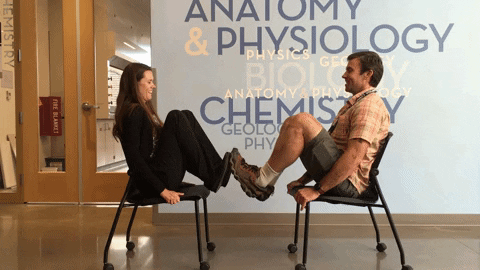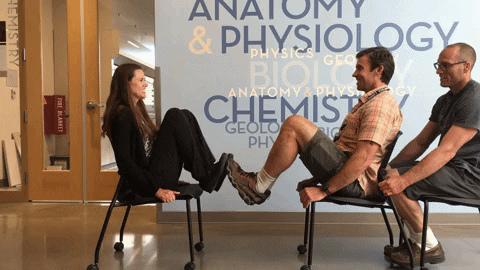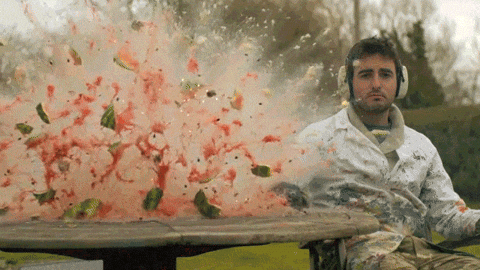# Center of Mass Velocity

If our lonely astronaut, introduced in the previous chapter, had something to push against then she could change her velocity.

Everyday Example: A two-body collision (explosion)

The following GIF shows a basic collision in which two objects push against one another and separate. In this example, the wheels minimize friction so that the two-person system is (almost) isolated (at least in the horizontal direction).Sitting in a wheeled chairs, Science Lab Technician Becky Kipperman (left) and Chemistry Professor Sean Breslin (right) push against one another. This collision causes a leftward change in velocity for Becky and a smaller rightward change in velocity for Sean.

Examining the previous example, you may have noticed that both Becky and Sean experienced changes in velocity, which is expected because each person experienced a force from an external object, which was the other person. You may have also noticed that Becky experienced a much greater change in velocity, which is also expected because she has a smaller mass than Sean. This effect is more apparent if when the difference in mass between objects is greater:

Everyday Examples: A two-body collision (explosion)

Initially this system is not moving. In order for the center of mass velocity of the isolated system to remain zero the different masses must experience different changes in velocity, which becomes more apparent when the difference in mass between the objects is greater:Sitting in a wheeled chairs, Science Lab Technician Becky Kipperman (left) and Chemistry Professor Sean Breslin (right) push against one another while the author is attached to Sean in another chair, but not touching the floor. This collision causes a leftward change in motion for Becky and a much smaller rightward change in motion for Sean and the author. Overall, the center of mass velocity of the whole system does not change.

We can predict the new velocities for Becky and Sean+Author by  recognizing that if we include all of them in our system from the beginning, then the forces between them become internal to the system. In that case the combined Becky + Sean + Author system is now isolated and that total system cannot experience a change in velocity. Making such a statement requires that we define what we mean by velocity of an entire system with multiple parts. The system velocity is defined center of mass velocity, (). You may remember that in Unit 4 we calculated the average location of all the mass in a system, which we called the center of mass. For that calculation we multiplied the mass of that piece by the location of that piece, added all those values up, and then divided by the total mass of the system.  Finding the velocity of the center of mass is very similar. For each part of the system we need to multiply the mass of that piece by the velocity of that piece, add all those values up, and then divide by the total mass of the system. For a two-body system like Becky and Sean there only two the calculation would look like this:

(1)The center of mass velocity of an isolated system cannot change so even if two components of the system collide the initial and finalvalues have to be the same:

(2)The bottom (denominator) of both sides of the previous equation are the same, so we can cancel it out:

(3)Now we see that saying the center of mass velocity of an isolated system cannot change is actually the same thing as saying the total of all the masses multiplied by their velocities can’t change. We can skip right to this step when solving problems and so call the combinationthe say that the total momentum of an isolated system cannot change.  That statement is known as the .

Everyday Example: Two-body collision

During the collision in the previous example we saw Becky left the collision with higher speed than Sean + Author. The combined mass of Sean + Author is roughly 3x Becky’s mass, so they should have 1/3 of her speed in the opposite direction. We can use the equation above to verify our intuition.

(4)The initial velocity of both system components was zero:

(5)Let’s have Becky be the first mass (). The second mass is 3x bigger, so:

(6)Dividing everything by:

(7)Moving Becky’s velocity to the other side

(8)We see that Becky’s velocity () is 3x bigger, which means that Sean’s velocity is 1/3 of hers. We also see that the velocities are in the opposite direction as indicated by one being positive and one negative. Everything checks out.

Reinforcement Exercises

# Conserving Momentum

We see now that the astronaut in our thought experiment could have thrown something, such as a wrench, in order to change her velocity. In that case she is interacting with another object. However, of the overall wrench + astronaut system cannot experience a change total momentum. That means the resulting velocity for the less massive wrench must be very large compared to the resulting velocity for the more massive astronaut.  This wrench-throwing mode of locomotion is really no different from you walking, but the situation is reversed in a way. When you push off the ground to walk forward you cause the Earth to move back in return. Essentially you are an astronaut using the Earth as a really big “wrench” to throw. The Earth is so big that it’s change velocity is not noticeable, while yours feels significant.

Everyday Example: Watermelon Explosion

Explosions must also obey the Law of Momentum conservation. If an object starts at rest, then when we add up the momentum of every piece of the object after the explosion we must end up with zero, just as in the previous examples of “explosions” with only two “pieces”.  Watching an explosion in reverse helps to illustrate this point:After the explosion of the watermelon the total momentum must be zero because it was zero before the explosion. The momentum of pieces moving in any one direction must cancel out the momentum of pieces moving in the opposite direction. The explosion is symmetric. (The table does redirect some pieces upward, so the explosion does not actually appear completely symmetric in the vertical direction.)

If we really pay attention to the watermelon explosion we can see that no pieces move downward because the table redirects them. If we were to actually add up all the momenta of all the pieces we would find that the watermelon system had a net upward . It appears that the momentum of the watermelon system alone was not conserved, but that is because it was not isolated. If we include the table and the earth that it’s attached to in our system so that the forces between them are internal and the new system is isolated, then account for the (immeasurable) downward velocity of the table and earth, we would find that the momentum of the system is conserved. In a later unit we will investigate how the forces between components in a collision are related to their individual changes in momentum.